# Efficient Radial FDTD Calculation in RSoft Photonic Device Tools

## Overview

It is well-known that FDTD (Finite-Difference Time-Domain) is the most rigorous numerical method for photonic device simulation. However, FDTD is also a very expensive approach in terms of both computer memory and computation time. Thus, its application is limited to very small structures only. Many photonic devices, such as diffractive lenses, are cylindrically symmetric. Utilizing a structure’s symmetry, a radial calculation can yield the same result as a full 3D simulation with a fraction of computer resources, as noted by the authors in the reference .

Fortunately, radial calculation was implemented in the very first version of the RSoft FDTD-based Photonic Device Tool, FullWAVE, in 2001. FullWAVE was the first FDTD simulation software in photonics field and over the last 2 decades, this feature has been used worldwide to simulate various cylindrical symmetric structures [2,3]. The accuracy and efficiency of the radial calculation, however, has never been systematically investigated.

In this application note, a detailed study on the radial FDTD calculation is made by comparing its accuracy and efficiency against a full 3D simulation. We show that this approach can also be applied to quasi-symmetric structures and gives very good estimation when compared to the accurate 3D results.

## Validation

In theory, radial calculation should give the same result as a direct 3D calculation for cylindrically symmetric structures since no physical approximation is made. In practice, however, different numerical errors could be introduced in different approaches. Therefore, a direct comparison is needed to validate the radial calculation.

## Device Layout

The example shown is a multi-level diffraction lens (MDL) similar to the one studied by the authors in reference .  Shown below is a MDL layout in RSoft CAD with designed focal length of 20µm and numerical aperture (NA) of 0.5. The cross-section of those rings cannot be viewed clearly in the 3D CAD layout and it is the same as the insert for the layout in 2D radial calculation.  Both were generated by Python script API, a new feature in the 2019.09 release. The pitch is 300nm for the sake of easy fabrication.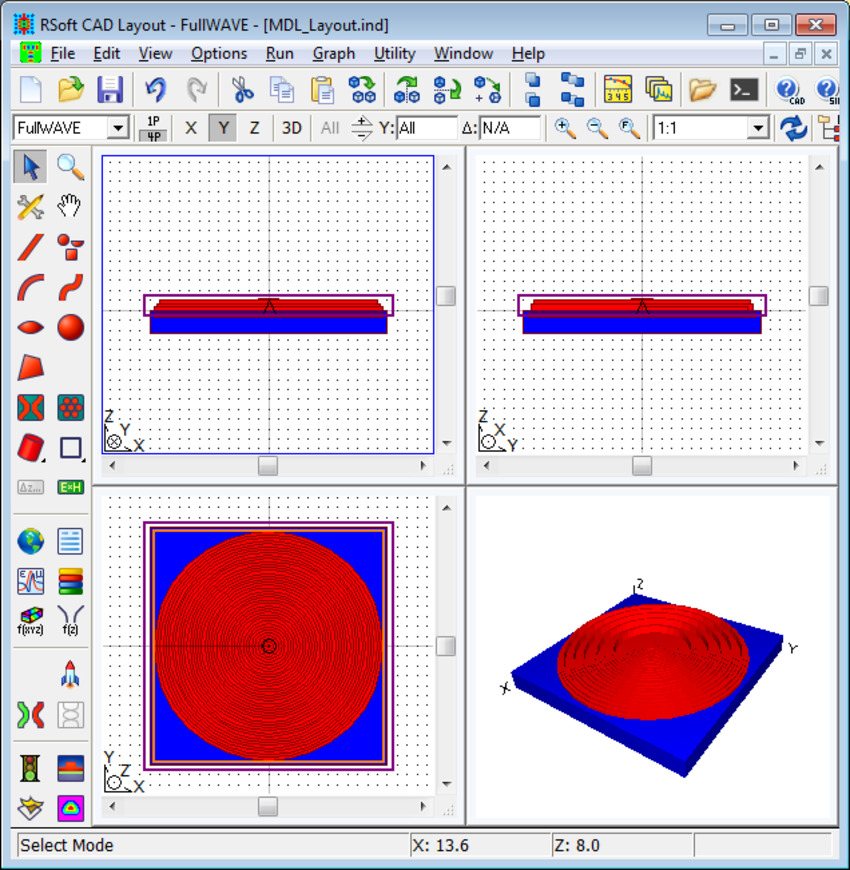Figure 1. Layout of the 3D MDL in RSoft CAD with insert for 2D radial calculation.

## 2D Radial Calculation

The simulation domain for this structure is 12.15µm X 26 µm with a mesh size of 10 PPW (Point Per Wavelength), i.e., 36nm in silica lens and 53nm in air.  Shown below, in Figure 2, are the calculation results. The focal length is F=20.1µm, which is very close the designed value F=20µm.

The simulation finished in less than a minute with only 0.04G RAM used.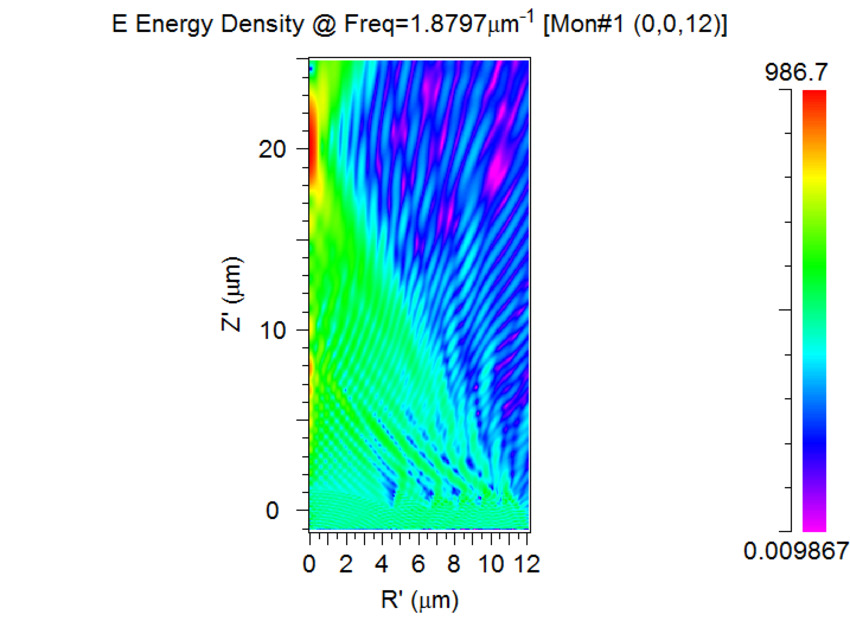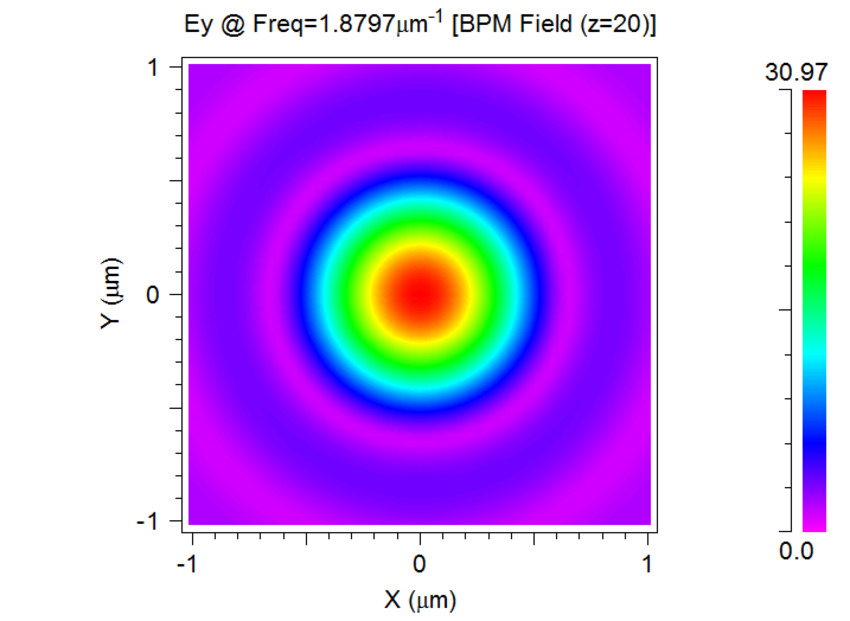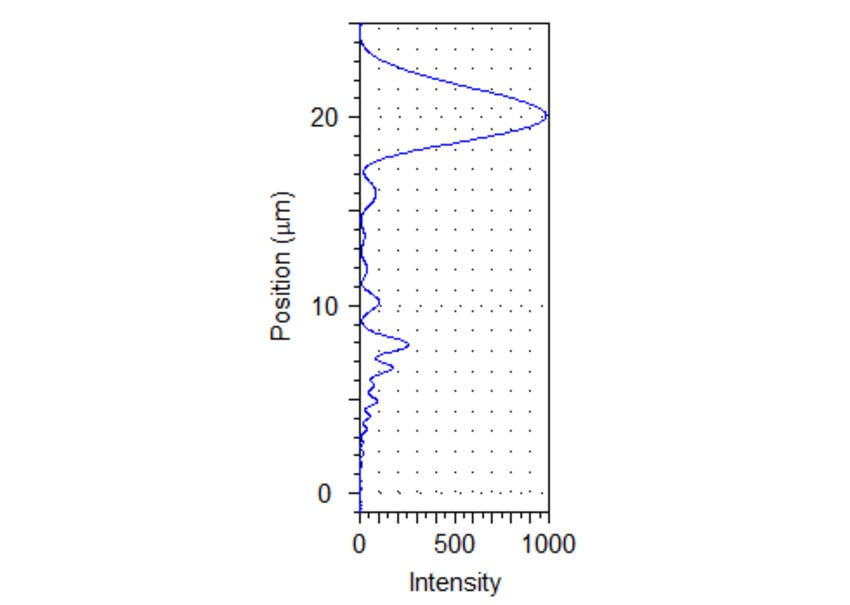Figure 2. 2D radial calculation results R-Z view (left); X-Y view at the focal point (Z=20µm) (center); intensity along the center (right).

## Full 3D Calculation

A 3D simulation is performed using the X- and Y-symmetry, so only a quarter of the lens is simulated. Shown below, in Figure 3, are the simulation results with a focal length of F=19.9µm, similar to the 2D radial result.

With the same mesh size, the 3D calculation took 21 minutes with about 10G RAM, significantly longer and using more RAM than the 2D radial calculation.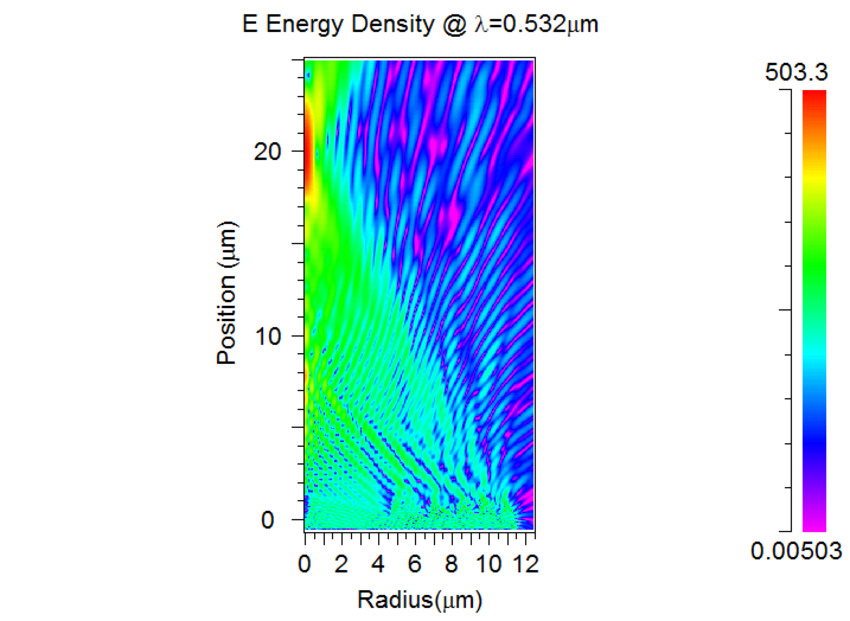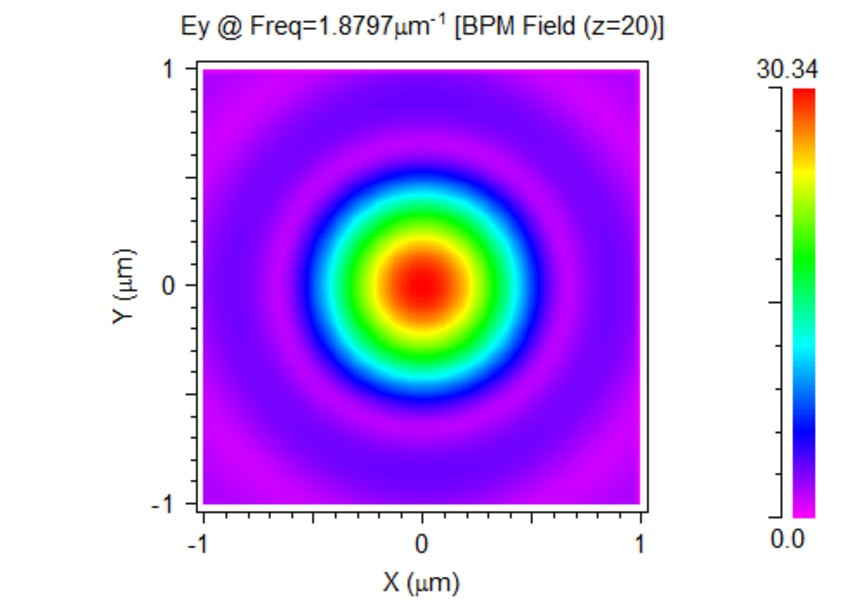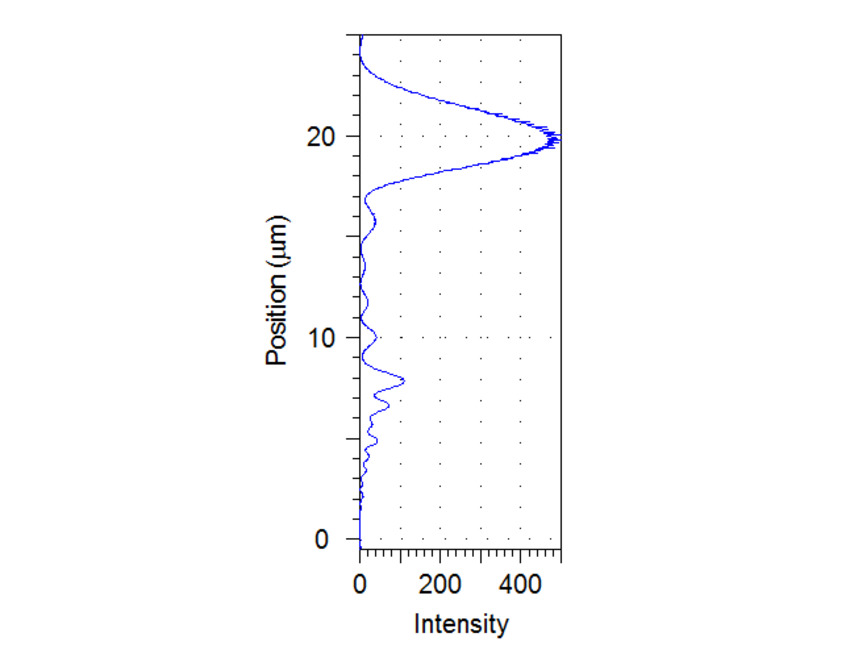Figure 3. Full 3D calculation results R-Z view (left);X-Y view at the focal point (Z=20µm) (center); intensity along the center (right).

## Comparisons

The above test case in Figure 3 shows that both the 2D radial calculation and the 3D calculation give almost the same results but use significantly different computation time and computer memory. To gain a general idea about the difference, a direct comparison is needed.

As we know, FDTD is a linear calculation, both computation time and computer memory are linearly proportional to the number of meshes and time steps. The total propagation time should be similar for 2D and 3D cases, except the time step is different by a factor of  , since the FDTD time step size is defined as: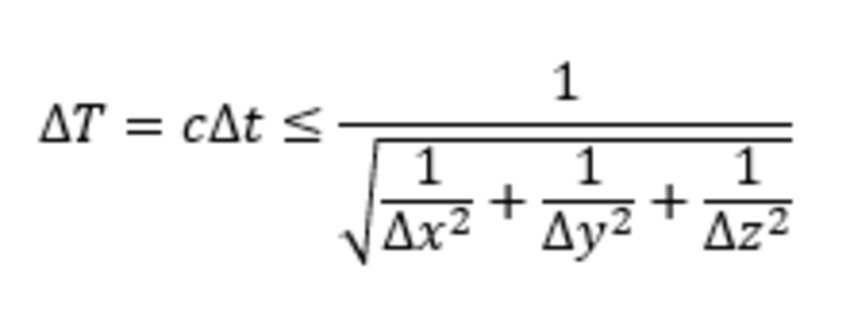To make an easy and fair comparison, we can simply change the mesh size instead of changing the physical dimension of the structure each time. An equal and uniform mesh size is used in all spatial directions.

Shown below, in Figure 4, are the comparison results for both computation time and memory as a function of mesh size.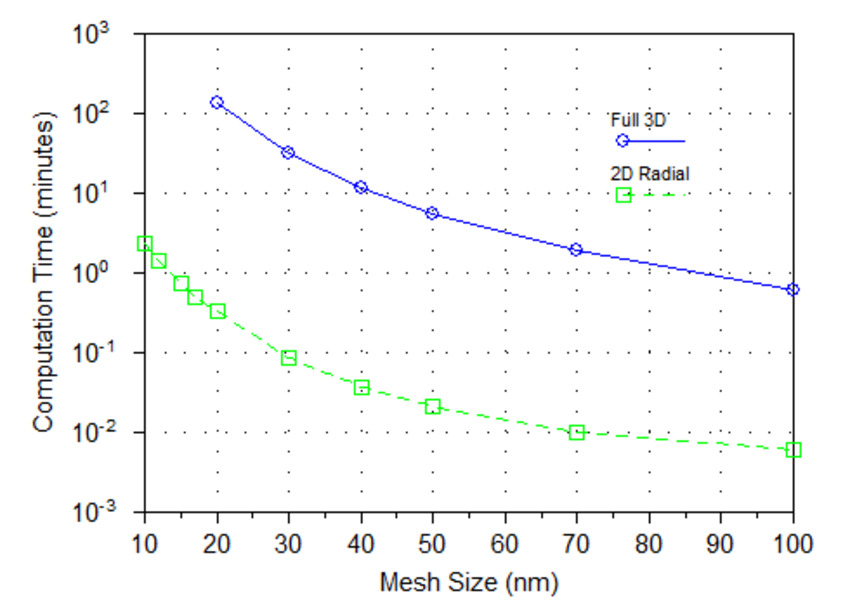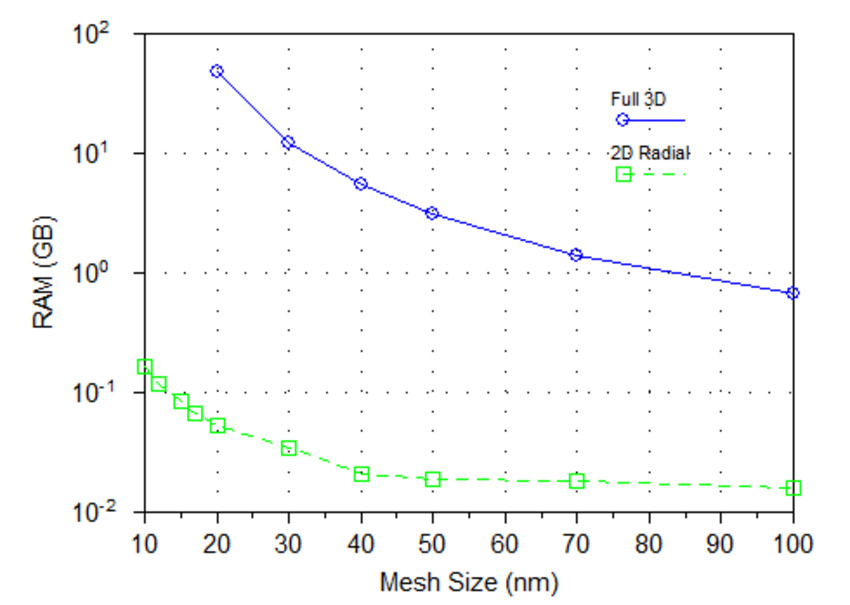Figure 4. Direct comparison on computer resource computation time (left); computer memory (right).

It is clear that the 2D radial calculation is several orders of magnitude faster than the full 3D calculation. The memory requirement is also several orders of magnitude less. The difference will be even more notable in larger structures.

## Quasi-Symmetric Structures

Although the radial calculation is valid for cylindrically symmetric structures only, it can also provide a quick estimation on some structure with cylindrically quasi-symmetric structures, especially when the real 3D solution is virtually impossible. Photonic crystal (PhC) for LED extraction enhancement is one example of this.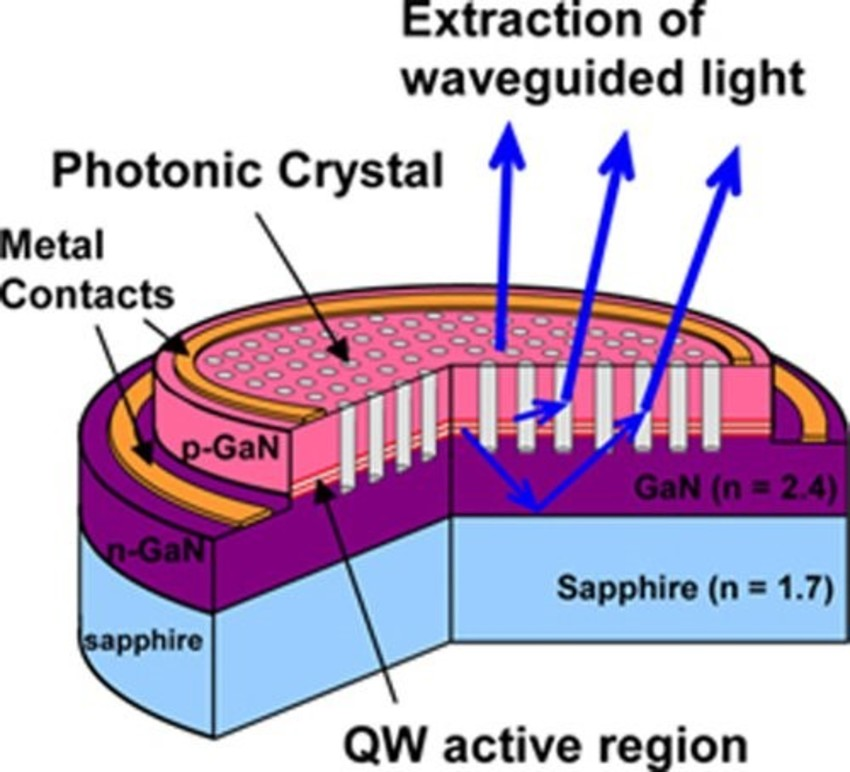Figure 5. Schematic diagram of a LED with photonic crystal.

For the structure shown above in 5 , the PhC creates a bandgap in the horizontal directions so that the light generated in the active layer has no other place to go, but to emit vertically.  Therefore, the PhC behaves like a Bragg grating and can be approximated by a circular Bragg grating for quick calculation, as shown below in Figure 6.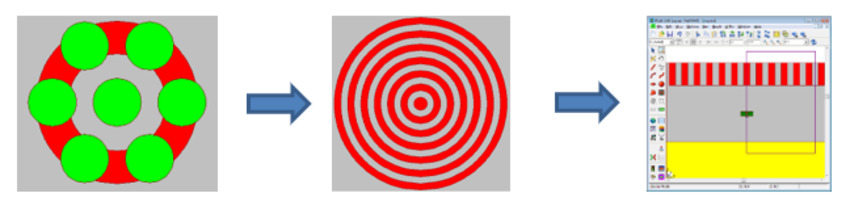Figure 6. Approximation of a PhC structure into a circular Bragg grating.

To validate the approximation, we performed an FDTD simulation, both 2D radial and full 3D, with RSoft FullWAVE and the simulation results are shown below in Figure 7.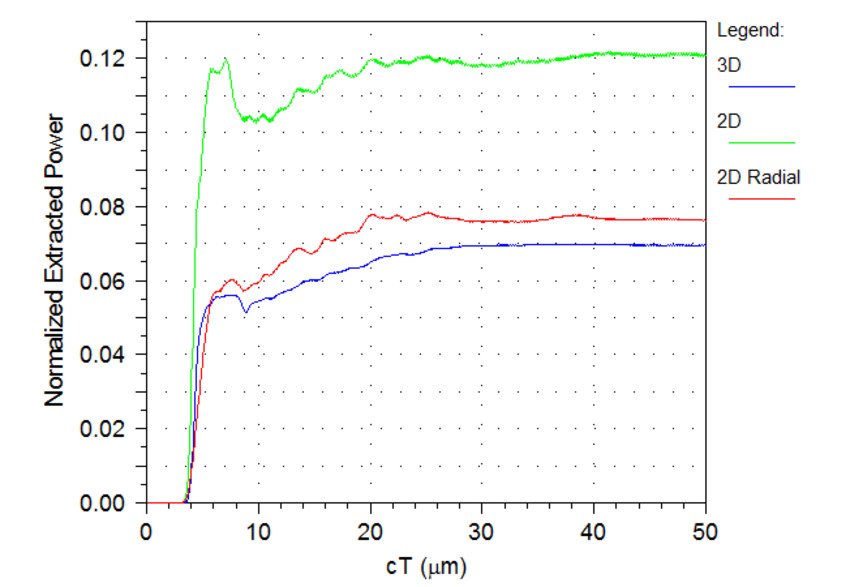Figure 7. Validation of 2D radial calculation for PhC structures.

The calculation results show that 2D radial calculation is a very good approximation to the full 3D calculation, while the saving on computer resources is tremendous, as we observed in previous sections.

Out of curiosity, we also did a 2D simulation and the result is significantly different simply because the 2D calculation turns the structure into 1D line gratings, which changes the structure completely.

## Summary

In conclusion, radial calculation in RSoft Photonic Device Tools provides an efficient and accurate way to simulate structures with cylindrical symmetry. It can save tremendous computer resources in terms of both computation time and computer memory. It also allows users to simulate larger structures which are virtually impossible for full 3D simulations.

In addition to cylindrically symmetric structures, the radial calculation can also apply to quasi-symmetric structures for a quick estimation, with some degree of error.

## References

 Banerji, S., Meem, M., Majumder, A., Vasquez, F. G., Sensale-Rodriguez, B., & Menon, R. (2019). Imaging with flat optics: metalenses or diffractive lenses. Optica, 6(6), 805-810.

 Kotlyar, Victor V., and Alexey A. Kovalev. "Nonparaxial propagation of a Gaussian optical vortex with initial radial polarization." JOSA A 27.3 (2010): 372-380.

 Yuan, G. H., S. B. Wei, and X-C. Yuan. "Nondiffracting transversally polarized beam." Optics Letters 36.17 (2011): 3479-3481.

 Barton, Daniel L., and Arthur J. Fischer. "Photonic crystals improve LED efficiency." SPIE Newsroom 10.1200603 (2006): 0160.